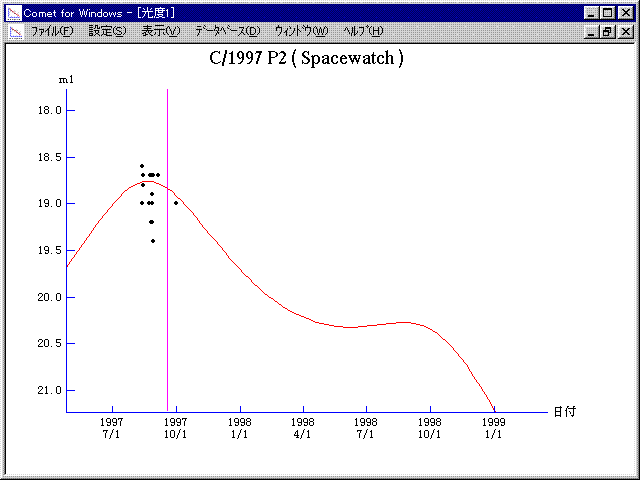# \$B%9%Z!<%9%&%)%C%AWB@1(B

C/1997 P2 ( Spacewatch )###\$B%W%m%U%#!<%k(B

 \$BH/8+F|(B 1997\$BG/(B8\$B7n(B12\$BF|(B \$BH/8+8wEY(B 18.6\$BEy(B \$BH/8+ Spacewatch (Kitt Peak)

###\$B50F;MWAG(B

```   The following improved orbital elements by Kenji Muraoka, are
from  90 observations  1997 Aug. 12 to Sept. 30, perturbations
by 9 Planets, Moon and 5 minor planets were taken into account.
The mean residual is +/- 0.63 arc seconds.

Epoch  =  1997 Aug. 20.0  TT       JDT = 2450680.5
T  =  1997 Aug. 31.12099       +/- 0.77531 (m.e.) TT
Peri. =   24.49517                +/- 0.18312
Node  =  302.91720                +/- 0.22980   (2000.0)
Incl. =   14.58375                +/- 0.05987
q  =    4.2633919              +/- 0.0011667 AU
e  =    1.0284013              +/- 0.0000916
1/a  =   -0.0066617              +/- 0.0000216 1/AU
orig. 1/a  =   -0.0000311
fut.  1/a  =   -0.0023503
```

###\$B@1?^(B1997\$BG/(B 5\$B7n(B 2\$BF|!A(B1998\$BG/(B10\$B7n(B24\$BF|(B

###\$B8wEYJQ2=(B

```        m1 = 10.0 + 5 log\$B&\$(B + 10.0 log r
```##### \$B50F;MWAG\$OB<2,7r<#;a\$N7W;;\$K\$h\$k\$b\$N\$G\$9!#(B \$B@1?^\$O(B StellaNavigator Ver.2.0 for Windows (\$B%"%9%H%m%"!<%D(B \$BJTCx(B / \$B%"%9%-!<=PHG6I4)(B) \$B\$G:n@.\$7\$?\$b\$N\$G\$9!#(B \$B8wEY%0%i%U\$O(BComet for Windows\$B\$G:n@.\$7\$?\$b\$N\$G\$9!#(B